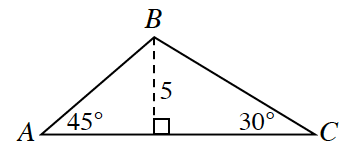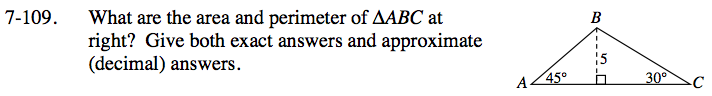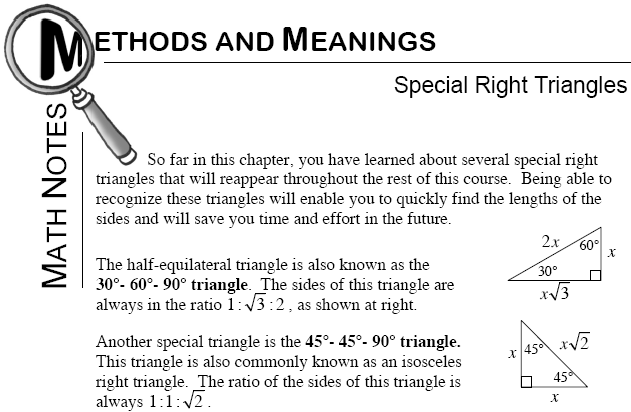### Home > INT3 > Chapter 7 > Lesson 7.2.5 > Problem7-109

7-109.
1. What are the area and perimeter of ΔABC below? Give both exact answers and approximate (decimal) answers. Homework Help ✎

2.The height creates two special right triangles. What are the 45º-45º-90º and 30º-60º-90º triangle patterns?

Refer to the Math Notes box below (from a previous course) to determine the unknown sides.
Then use this information to calculate the area and perimeter of the original triangle.